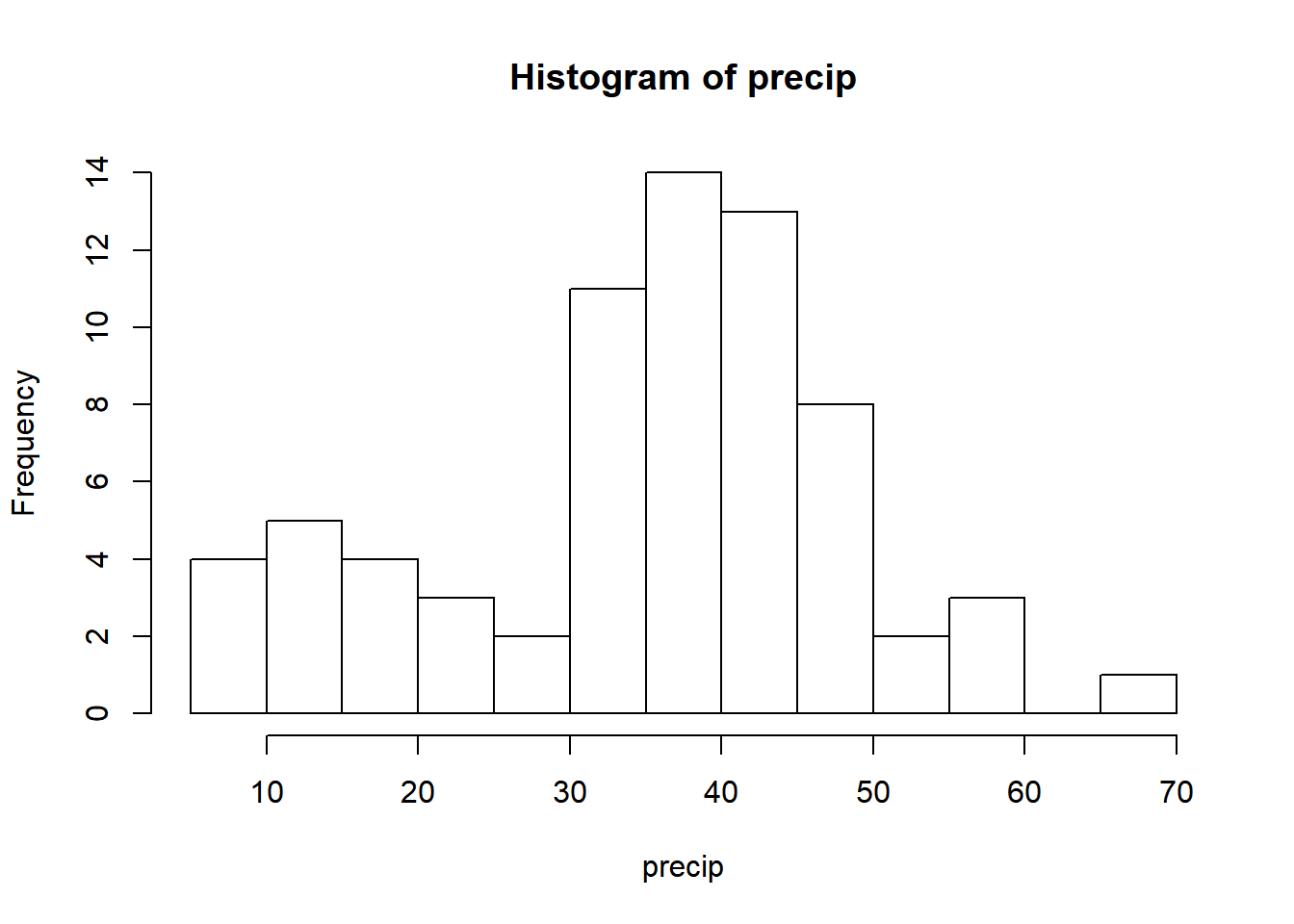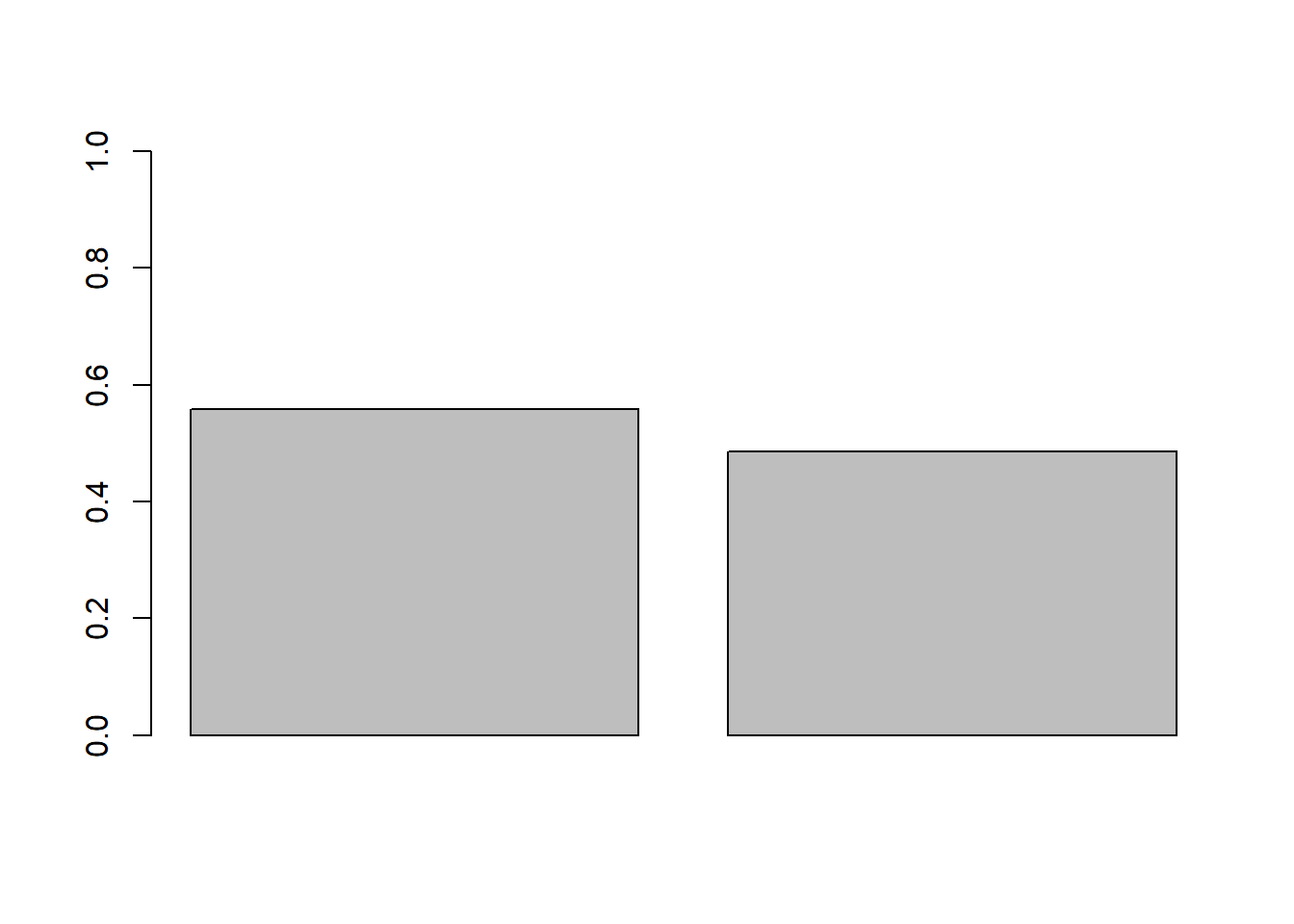### Homework exercise

To be solved at home before the exercise session.

1. A simple sample size calculation can be performed for binary proportion confidence intervals as follows. We bound the standard deviation estimate from above as $$\sqrt{\hat{p}(1 - \hat{p})} \leq 0.5$$ to obtain the conservative confidence interval, $\left( \hat{p} - z_{\alpha/2} \frac{0.5}{\sqrt{n}}, \hat{p} + z_{\alpha/2} \frac{0.5}{\sqrt{n}} \right).$ The half-width of a confidence interval is known as its margin of error and for the conservative confidence interval the margin of error does not depend on the proportion of “successes”. Thus we can compute a universal sample size for which a certain desired margin of error is reached.
1. Compute the required sample sizes to obtain the margins of error of 0.01, 0.02 and 0.03 for a 95% conservative confidence interval.
2. Study how much the calculations in part i over-estimate the required sample sizes when the proportion of successes is small $$\hat{p} = 0.05$$. That is, redo part i using the regular binary confidence interval in slide 4.6.
# i. The half-width of the conservative 95% interval is 1.96*0.5/sqrt(n). This equals 0.01*a if
# 1.96*0.5/sqrt(n) = 0.01*a <=> 1.96*0.5/(0.01*a) = sqrt(n) <=> n = 9604/a^2.
# That is, the required sample sizes are n = 9604, 2401, 1068.
#
# ii. The half-width of the standard 95% interval for \hat p = 0.05 is 1.96*sqrt(0.05*0.95)/sqrt(n). As in part i, we obtain n = 1824.76/a^2 and the true required sample sizes are 81% (= 1 - 1824.76/9604) smaller than those approximated in part i.
b. A manufacturer claims that only 6% of their products are faulty. To investigate this, a customer picks a random sample of size $n$ of products and observes the proportion of faulty ones to be $\hat{p} = 0.09$. He tests the manufacturer's claim using the asymptotic one-sample proportion test in slide 4.9. Is the p-value of the test smaller for sample size $n = 100$ or $n = 200$?
# The Z-value of the test is proportional to the square root of the sample size n. Thus increasing the sample
# size increases the Z-value and consequently pushes it towards the tail of the distribution, decreasing the
# p-value. Thus the p-value for n = 200 is smaller
#
# An intuitive reasoning for the result is that the difference between 0.06 and 0.09 is "proportionally"
# larger for n = 200 than for n = 100 (as larger n implies increased accuracy) and as such also more
# deviating.

### Class exercise

To be solved at the exercise session.

Note: all the needed data sets are either given below or available in base R.

1. The data set precip describes the average annual amounts of precipitation (rainfall) in inches for 70 United States (and Puerto Rico) cities. A city is said to be dry if its average annual rainfall is less than 20 inches. Treat the data as a random sample amongst all US cities and estimate a confidence interval for the proportion of dry cities in the US.
1. Visualize the data.
2. Create a new variable which takes the value 1 if the city is dry and 0 otherwise.
3. Compute an approximate 95% confidence interval for the proportion of dry cities.
4. What is the interpretation of the confidence interval in part c?
# a.
# Distribution seems to be bi-modal.
hist(precip, breaks = 10)# b.
precip_dry <- 1*(precip < 20)
precip_dry
##              Mobile              Juneau             Phoenix
##                   0                   0                   1
##         Little Rock         Los Angeles          Sacramento
##                   0                   1                   1
##       San Francisco              Denver            Hartford
##                   0                   1                   0
##          Wilmington          Washington        Jacksonville
##                   0                   0                   0
##               Miami             Atlanta            Honolulu
##                   0                   0                   0
##               Boise             Chicago              Peoria
##                   1                   0                   0
##        Indianapolis          Des Moines             Wichita
##                   0                   0                   0
##          Louisville         New Orleans            Portland
##                   0                   0                   0
##           Baltimore              Boston             Detroit
##                   0                   0                   0
##    Sault Ste. Marie              Duluth Minneapolis/St Paul
##                   0                   0                   0
##             Jackson         Kansas City            St Louis
##                   0                   0                   0
##         Great Falls               Omaha                Reno
##                   1                   0                   1
##             Concord       Atlantic City         Albuquerque
##                   0                   0                   1
##              Albany             Buffalo            New York
##                   0                   0                   0
##           Charlotte             Raleigh             Bismark
##                   0                   0                   1
##          Cincinnati           Cleveland            Columbus
##                   0                   0                   0
##       Oklahoma City            Portland        Philadelphia
##                   0                   0                   0
##           Pittsburg          Providence            Columbia
##                   0                   0                   0
##         Sioux Falls             Memphis           Nashville
##                   0                   0                   0
##              Dallas             El Paso             Houston
##                   0                   1                   0
##      Salt Lake City          Burlington             Norfolk
##                   1                   0                   0
##            Richmond      Seattle Tacoma             Spokane
##                   0                   0                   1
##          Charleston           Milwaukee            Cheyenne
##                   0                   0                   1
##            San Juan
##                   0
# c.
# Mean of the dummy variable gives the proportion
phat <- mean(precip_dry)
n <- length(precip_dry)

# The approximate 95% CI
ci <- c(phat - 1.96*sqrt(phat*(1 - phat))/sqrt(n), phat + 1.96*sqrt(phat*(1 - phat))/sqrt(n))

# d.
# For every 100 random samples of US cities of size 70, in roughly 95 of them the confidence interval
# computed as in part c contains the true proportion of dry cities in the US. We hope that the single
# interval we have is one of these 95.

1. In 2018, a proportion $$p_0 = 0.098$$ of people living in Finland had their last name beginning with a vowel. Treat the previous fact as a hypothesis and test it using the participants of the exercise session as a sample.
1. Observe the sample size $$n$$ and the observed proportion $$\hat{p}$$ of participants having last names beginning with a vowel.
2. Write down the assumptions and hypotheses of the one-sample proportion test.
3. Conduct the test, using the exact version of the test if the requirements of the approximative test on slide 4.9 are not fulfilled.
4. What is the conclusion of the test? Can this conclusion be taken as evidence against/for the “hypothesis”?
# a.
# Substitute the real values in place of the defaults:
n <- 35
x <- 5
phat <- x/n

# b.
# Assumptions:
# The sample is iid from Bernoulli with parameter value p where p is the proportion of people living in
# Finland with last name starting with a vowel.
# (that is, everyone has their last name beginning with a vowel with equal proability and independently
# of each other)
#
# Hypotheses:
# H0: p == 0.098
# H1: p != 0.098

# c.
# Exact test if n*phat <= 10 or n*(1 - phat) <= 10
binom.test(x, n, p = 0.098)
##
##  Exact binomial test
##
## data:  x and n
## number of successes = 5, number of trials = 35, p-value = 0.3855
## alternative hypothesis: true probability of success is not equal to 0.098
## 95 percent confidence interval:
##  0.04806078 0.30257135
## sample estimates:
## probability of success
##              0.1428571
# Asymptotic test else
prop.test(x, n, p = 0.098, correct = FALSE)
## Warning in prop.test(x, n, p = 0.098, correct = FALSE): Chi-squared
## approximation may be incorrect
##
##  1-sample proportions test without continuity correction
##
## data:  x out of n, null probability 0.098
## X-squared = 0.79671, df = 1, p-value = 0.3721
## alternative hypothesis: true p is not equal to 0.098
## 95 percent confidence interval:
##  0.06260231 0.29375554
## sample estimates:
##         p
## 0.1428571
# d.
# Substitute conclusions here. The conclusions can most likely not be used to draw inference on the
# proportion of people in the whole Finland as the session participants make a poor *random* sample of this population.
# At best, the participants could be considered a random sample of all Aalto students in
# particular programmes.

1. In the beginning of the year a total of $$n_1 = 963$$ people were polled and $$x_1 = 537$$ out of them expressed their support for a certain presidential candidate. In a poll organized one month later $$x_2 = 438$$ people out of $$n_2 = 901$$ people claimed to support the candidate. Based on the data, has the support for the candidate decreased?
1. Visualize the data.
2. Write down the hypotheses for a two-sample proportion test and conduct it on a significance level 5%.
3. What are the conclusions of the test?
4. What assumptions were required by the test in part b? How can a poll-organizer ensure that they are satisfied?
# a.
x_1 <- 537
n_1 <- 963

x_2 <- 438
n_2 <- 901

phat <- c(x_1/n_1, x_2/n_2)
barplot(phat, ylim = c(0, 1))# b.
# H0: p_1 = p_2
# H1: p_1 > p_2
# where p_1, p_2 are the success probabilities (probabilities to support the candidate) in the two samples
# which are assumed to be independent of each other and iid from two Bernoulli distributions.

prop.test(c(x_1, x_2), c(n_1, n_2), alternative = "greater", correct = FALSE)
##
##  2-sample test for equality of proportions without continuity
##  correction
##
## data:  c(x_1, x_2) out of c(n_1, n_2)
## X-squared = 9.5406, df = 1, p-value = 0.001005
## alternative hypothesis: greater
## 95 percent confidence interval:
##  0.0335168 1.0000000
## sample estimates:
##    prop 1    prop 2
## 0.5576324 0.4861265
# c.
# The one-sided p-value ~= 0.001 < 0.05 -> we reject the null hypothesis in favor of the alternative.
# That is, the support has decreased.

# d.
# (The assumptions are stated above in the answer to b.) To ensure that the samples are independent and iid
# and representative of the nationwide support level, the pollmaker should draw the samples perfectly randomly
# from amongst all eligible voters.

1. (Optional) Find out how the Wilson score confidence interval for a binary proportion is computed and locate an R package which computes it (there are several). The Wilson interval gives a better coverage probability than the standard CI given in slide 4.6 for small sample sizes. Find out how large this improvement is by conducting a simulation study. For example, simulate m = 10000 samples from the binomial distribution with n = 15 and p = 0.3 and compute for both intervals the proportion of the samples in which the interval contains the true parameter value.